5th April 2021

Introduction to Tesla coils

What is a Tesla coil

Tesla coil is simply put a resonant amplifier with an air core transformer. It steps up the input voltage by orders of magnitude, to so high voltages that the electrons on the secondary circuit repel each other with sufficient force to escape into free air by ionization. That's the idea behind throwing arcs into surrounding air. Now you might ask, why we call it a coil when it is in fact a whole transformer?

Tesla coils are characteristic with their large secondary coil with thousands of windings and a primary coil with very low windings count, usually less than 10 windings are used on the primary side. Unlike the primary coil, you cannot overlook the secondary, and this is why we call it incorrectly a "coil".

Nikola Tesla's original idea behind a Tesla coil

There are many misconceptions about the work of Nikola Tesla, as a common example; people often say that Tesla wanted to create a device which would serve as an unlimited free energy source, there are even rumors that he created a death beam which could destroy whole cities across continents. Of course, those are only misconceptions and foolish rumors. Now why there are so many fake news about Tesla? He did extraordinary work in the field of electromagnetism, thanks to Tesla we have AC motors and very efficient power distribution and much more, but he also worked on wireless energy transfer device, which we today call a Tesla coil. Many of his projects were a big success but his dream about wireless power transfer was simply unrealistic. Transmitting power over a long distance with a Tesla coil is impossible even with today's technology. The problem with this energy transfer is that the electromagnetic field created by the coil quickly decays in amplitude as you go further away. Also the power is not directed, it is emitted in all directions. Consider our sun, and how little of its energy we are able to catch here on Earth, it's pretty much the same story with a Tesla coil. We could build a very large Tesla coil but even with our modern technologies and efficient power switching electronics, the efficiency of such device would be terrible. Despite the obvious problems with such device, Tesla continued his work at his Wardenclyffe laboratory. As there was no success, Tesla's sponsors didn't want to financially support him any longer. Tesla afterwards proposed many ideas to the military, for example a death rays, weapons and free energy harvesters. He got financed again and used this money to continue on his work with wireless power transfer. Later the US military and authorities found out, he was not using the money for the proposed ideas and his work got confiscated.

But his idea was not useless at all. Although we cannot transfer power this way, we can at least transfer information. The first spark-gap transmitters were based on the idea of a Tesla coil and today we are able to transfer energy wirelessly even with our mobile phones. Who knows when we would be able to like photos on Instagram or send a message on Messenger if it was not for Nikola Tesla and his Tesla coil.

Resonance

Resonance is a physical phenomena which is literally all around us. Resonance is when a system's amplitude reacts way more when excited by a certain frequency. We call this frequency a resonant frequency or natural frequency or sometimes we also call it "the fundamental". You can see this behavior on the following graph:In real world most systems have more than just one resonant frequency, usually the system also responds to multiples of this frequency. We'll address this issue later, but let's talk about practice for a while. What is a common example of resonance?

Let's consider musical instruments; imagine a guitar string, what determines which frequency will we hear if we play the string?

We can approximate the frequency as follows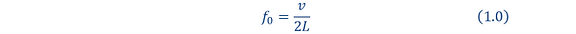where f0 is the natural frequency, v is the speed of sound and L is the length of the string. Note that the speed of sound in the string varies with mass and tension and many other factors. This makes sense if you consider a guitar which has all of its strings the same length, yet all the strings have different fundamental. This is because the speed of sound in the string varies as the strings have different mass and tension. You play the guitar by hitting the strings, when you hit the string, it vibrates at it's natural frequency, and that is the tone you hear. You can of course play many more frequencies as you press fingers on the near end of the string, you make it effectively shorter, thus the length of the string becomes smaller and the resonant frequency becomes higher, so you hear a higher tone. This is the idea behind all string instruments. Ever wondered why pianos have the shape they have?The shape of the piano has it's purpose. Notice how the piano is longer on the left side, that's where you play your low notes. Now look on the right side, where the piano is quite short. This is the area where you play higher notes. With higher frequencies the piano string gets shorter and that's why the shape of the piano is what it is.

Remember how I wrote that a system can have more resonant frequencies? Let's imagine a string again; the string does not only have a length, it also has some width and height. And if you somehow excite a string to vibrate not on it's length but on its width, you could apply the same formula to calculate its resonant frequency again. It may be easier to visualize on a following rectangular object:If you excite the vibrations on the longer side of the rectangle then the frequency you would hear would be equal to v/2b while if you do it on the shorter side, you would hear v/2a. When hitting a guitar string, you actually excite all the fundamental frequencies, but the higher fundamentals are beyond your hearing capability (human ear can only hear certain frequencies; usually in the range 20Hz - 20kHz).

In real world, systems usually have way more fundamentals, since no object is a perfect rectangle or a sphere or a string. In fact, every object in the world is not perfectly shaped as there are always some imperfections.

Resonance is something we can't overlook when designing a system. There is a very famous example of what the consequences can be; Tacoma Narrows bridge. Tacoma Narrows bridge was built in 1940 and it only lasted for 4 months before it collapsed. The engineers didn't take into account the resonant frequency of the bridge. When the weather was windy, the bridge often wiggled and vibrated, that's because the wind hitting the bridge excited it's natural frequency and in November of 1940 when the wind was really fast, the bridge wiggled so much that it eventually collapsed. There is a famous video of the bridge collapsing that's worth the watch:

Resonance in electrical systems

What we have discussed up until now was a resonance in mechanical systems, let's now discuss resonance in electrical systems. And no, resonance in electronics doesn't mean that our circuits vibrates along their axis, in electricity it's the voltage and current that oscillate. Before we continue, it's worth the note that these explanations are very simplified. Voltage and current can oscillate in any circuit which has a capacitance and an inductance thanks to the properties of capacitors and inductors. Any capacitor will always prevent a change in voltage and any inductor will resist a change in flowing current. What this actually means is that it takes time before the voltage on a capacitor rises to the supplied voltage. When the voltage on the capacitor is equal to the supply voltage, we say that the capacitor is charged. And similarly a current in an inductor cannot instantly change, it rises until it reaches maximum amplitude. When the current flowing through an inductor is at its maximum, we say that the inductor is charged. Now imagine a scenario where we have a circuit which only consist of capacitor and an inductor. We call this circuit an LC circuit.Now imagine that we charge the capacitor to its maximum voltage and we keep the inductor current at 0 and let's also plot the voltage of the capacitor and current in the inductor in timeYou can see that the current

through the inductor is 0 and the voltage of the capacitor is at its maximum, now let's "play" this simulation to see what will happen

What's going to happen is that the capacitor will discharge into the inductor, its voltage will slowly fall while the inductor current will rise.The capacitor voltage has dropped to 0 as it discharged into the inductor, while inductor's current is now at maximum and it's fully charged. Now we have a following situationNow what's going to happen, is that the inductor wants to keep the current flowing, this way it will discharge into the capacitor and the capacitor will charge. Pay attention to the polarities. The current is flowing from + of the capacitor to its -. That's why voltage polarity of the capacitor will be reveresed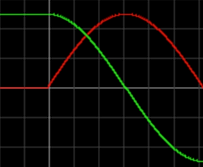The capacitor voltage is now at its maximum in the opposite polarity while the current flowing in the inductor is at zero.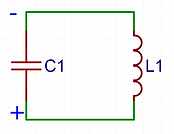You can probably guess what will happen now; the capacitor will discharge into the inductor, as before but this time in the opposite polarity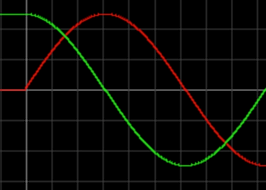In the next step we will get back to the start. Inductor is now charging the capacitor to it's original polarity while the inductor discharges to 0.And we're back where we started. The capacitor voltage is on its maximum in the original polarity and the inductor current is at zero. You can now see those beautiful sinusoidal oscillations of voltage and current.Notice how the frequency is fixed at all times. This is the resonance frequency of the LC circuit which does not change, unless you change the capacitance or the inductance in the circuit. Of course in reality, the oscillations do not have the same amplitude unless you keep pushing energy into the system. More realistic waveform would look like this:6th April 2021

This happens because the energy in the system is lost due to resistances in the circuit. Electrical energy is converted into heat on resistive elements.

How do we make use of it?

Consider following circuits:Two LC circuits, one is being driven at it's resonant frequency as it is emitting electromagnetic fields captured by the second LC circuit. The 2nd LC circuit acts as a receiver and for the optimal energy transfer, we want its resonant frequency to match the transmitter resonant frequency. If we transfer energy at a different frequency, the energy transfer will be poor and we will be lucky if we even catch any energy with the receiver. How so? Well to explain that, we have to talk about impedance.

Impedance is an overall effective resistance of a circuit with capacitors and/or inductors. Capacitors and inductors have a property called Reactance. Reactance is telling us how much do the capacitive/inductive elements in the circuit oppose the flow of current. Reactance is basically an "AC resistance". Impedance is simply a real resistance of the circuit together with the reactance and we can easily calculate it:Where |z| is impedance, X is the reactance and is of course the resistance. Now how do we calculate the reactance? Well we have 2 types of reactance. A capacitive reactance X_C, created by the capacitive elements in the circuits and an inductive reactance X_L, created by the inductive elements. We can calculate both:From which we can calculate the overall reactance as follows:As you can see from the formula 2.1 above, the inductive reactance increases with frequency. The faster the current tries to change, the harder the inductor fights back. At direct current which is effectively "zero frequency", the inductor acts as a short circuit, while if we would increase the frequency to infinity, it's reactance would also be infinite. Capacitors act the opposite way, they block DC currents (infinite reactance at DC), while the higher the frequency the lower is the reactance.

But let's now focus on the equation 2.3. What would happen if we had a following circuit:Let's assume the R1, L1 and C1 are fixed values and that we can change the frequency of the U1 AC voltage source. What if we use such a frequency that would make X_L equal to X_C?

We can always find such a frequency, we only need to solve the following equationWe can find this frequency for any circuit with capacitance and inductance! And as you probably already guessed, this frequency is the resonant frequency of the circuit. So what happens if we drive such a circuit at it's resonant frequency? Well at resonance, the capacitive reactance and the inductive reactance are equal and if we apply formula 2.3 to calculate overall reactance, we get 0. Now if we want to calculate the impedance of the circuit we find out that at resonance, the impedance is only equal to the resistance of the circuit. So at resonance, only the resistance of the circuit can limit the flowing current.

Let's go back to our transmitter-receiver circuit, but this time we'll make some changes. Let's assume that the transmitter is transmitting a signal at a frequency of 100MHz, and we want to have maximum possible current induced in the receiver. Let's also introduce some resistance into the circuit and let's add some real world values.You can find similar circuit inside a car radio, where you have a fixed inductance and a variable capacitor, which you tune to catch the frequency you want.

Let's make some numerical example but first we have to simplify some things to make it easy to understand. We will assume that the voltage we induce on the receiver will not change with the frequency, it will always be exactly 1V. Let's also assume that the only losses in the circuit are caused by the resistance R1.

Now let's count. When will the flowing current be at maximum? At resonance, of course, as the reactances will cancel each other out and the only thing left to limit the current will be the resistor R1. We can also ask a question: are we even able to tune this circuit to 100MHz? Well let's try it by substituting the values of the L1 inductance of 1uH and the maximum/minimum variable capacitor capacitance into equation 2.8With the current capacitor we have a frequency range from 15.92MHz up to 159.16MHz, so yes we will be able to tune it to 100MHz. Now let's calculate the needed capacitance for 100MHz signal by solving the equation 2.4 for capacitance:The needed capacitance is 2.533pF. At this capacitance, the inductive and capacitive reactance will cancel each other out and the current flow will be only limited by the 100 Ohm resistor R1. Since we made an assumption that the induced voltage in the receiver is 1V, then the current flowing will be 1V divided by 100 Ohms which gives us 10mA. Now let's play around a bit. What current would be flowing if we were just slightly off resonance? Let's say we put our capacitor value to 3pF. New resonant frequency would be 91.88MHz, we're not even 10% off the 100MHz signal. Let's calculate the current now. This time we have to calculate the reactance, because since we're off the resonance, they will not cancel each other anymore. So let's calculate:Notice how the inductive reactance is higher than capacitive. When this happens, we say that the circuit has an inductive character. And vice versa if the capacitive reactance is higher than inductive reactance, we say that the circuit is more capacitive. Now let's calculate total impedance and the flowing current:Consider that we are only 8.2% off the resonance and the flowing current dropped almost by 30%!. If we were only 20% off resonance, the flowing current would drop by more than 60%. So now you are able to appreciate that being even slightly off the resonance, the energy transfer gets very poor. Keep in mind that this example was grossly simplified.

Resonant voltage/current rise

Resonance has another amazing property. In resonance, the energy of the system is stored in the oscillations and is not lost. Thanks to this, if we push more energy into the system, it will add to the energy which is already in the system. This way we can supply a resonant circuit with some voltage but thanks to already existing energy in the circuit, the voltage will rise even above our supply voltage. And we get something we call a resonant voltage rise. Basically our voltage increases with every cycle indefinitely!Of course in real life, we will never have resonant rise which would climb in amplitude into infinity, there are always some energy losses which eventually stabilize the system into equilibrium. This also works in classical mechanics, and it is the reason why resonance can be rather destructive.

Spark gap Tesla coil (SGTC)

Now we have all the theory needed to discuss the working principle of the first ever Tesla coil design, which is the "Spark gap Tesla coil".

As already mentioned previously, a Tesla coil is actually a transformer, but not your ordinary transformer. Tesla coil uses resonance for optimal energy transfer between primary-secondary and it develops resonant voltage rise. Transformers consists of primary coil with N1 windings and secondary coil with N2 windings. In a basic transformer a simple formula can be applied to calculate the voltage on the secondary if you know your supply voltage:In a Tesla coil transformer, it is just a bit more complicated.The output voltage of the Tesla coil is also dependent on so called Q-factor. We often say Q-factor stands for the Quality of resonance. The higher your Q-factor is, the higher amplitude oscillations you will get, in this case higher voltage. On the other hand, higher the Q, the harder it is to tune your circuit to resonance.A Tesla coil is usually a pretty "High-Q" system, meaning that is has to be precisely tuned to the resonant frequency. Once the coil is tuned, the voltage rise on the secondary coil is huge and the voltage can rise to many hundreds of kV up to a few MV.

Let's now finally draw a schematic of an SGTC.Let's now discuss step by step what is happening in such a circuit. First we step up mains voltage by a basic transformer, so we get a high voltage on the primary side. This high voltage will slowly charge the resonant capacitor C1. We have to make sure that the spark gap will trigger once the capacitor is charged. The spark gap is basically voltage controlled switch, once the voltage on it is sufficient enough to breakdown the air between its electrodes, the spark gap triggers and a spark is created. The spark consist of high temperature plasma which is a low resistance path. When the spark gap triggers it basically shorts C1 into the primary coil of T2. As you may noticed already, the primary coil together with C1 makes up a resonant circuit. Once the spark gap triggers, they will oscillate together until they lose all the energy due to resistances and energy transfer to secondary coil.During the oscillations a very high currents flow through the primary coil, hundreds even thousands of Amperes. These high power oscillations occur every time the capacitor charges to spark-gap trigger voltage no matter the polarity. Theoretically if our mains voltage has a frequency of 50Hz, we should see the oscillations 100 times a second (since the capacitor gets charged twice during one sine wave period). If we now tune our secondary resonant circuit to match the frequency of these oscillations and the number of turns on the secondary is way higher than on the primary then we will get oscillations of very high voltage on the secondary. So what do we need to do? We need the following expression to be true:Once these conditions are satisfied, the voltage on the secondary during these oscillations can rise to mega Volts! And this is how we can create large artificial lightning.Nikola Tesla and his spark gap Tesla coil shooting huge streamers10th April 2021

For more detailed explanation I highly recommend to watch DiodeGoneWild's YouTube series on spark gap Tesla coil.

Rotary spark gap Tesla coil (RSGTC)

The classic spark gap has quite a few problems. The arc may not quench, it can overheat quite easily and it usually is not capable of running the coil for too long. This can be partially fixed by making so called RSGTC. RSG is just a spark gap which electrodes are placed on a spinning wheel.

This design has many advantages; you can place more electrodes on, which helps with heat dissipation and wear out. You can adjust the RPM which changes the firing frequency of the sparks. As the electrodes are moving away from each other, the arcs will definitely quench. Although there is a problem with this forced quenching. If you force an arc to quench, you can quench it when the maximum current is flowing, this creates massive change or current which will induce a very high voltage spike in your primary circuit. This voltage spike will be distributed on your mains power transformer, and it can and will destroy it. This is the reason why usually a rotary spark gap has another classical safety spark gap in parallel, to clamp the voltage of the occasional spikes.

RSGTC topology is usually suitable for high power spark gap tesla coils. They are usually actively cooled with a fan blowing cold air on the electrodes, helping with heat dissipation and arc quenching.A rotary spark gap design5th August 2021

Solid state Tesla coil (SSTC)

In the late 20th century transistors and other switching electronics got cheap enough so any electronics hobbyist could buy them. This started a new era of solid state Tesla coils. The problem with SGTC/RSGTC is that we cannot really control it anyhow. The sparks fly out at pretty unpredictable frequency (there are some designs of rotary spark gaps which can solve this issue). The spark gap is also very loud and causes a very high electromagnetic interference which can easily destroy nearby electronics. The efficiency a spark gap is also often quite questionable. In modern days, we like to design electronics so that we have a full control of its operation. We want to change the spark frequency and output power on demand, we want to control it with a microprocessor or a logic circuitry.

While in SGTC the coil resonates on it's own when the spark gap triggers, with a semi-conductors we have to drive the coil very precisely. But how do we even force an AC current to flow with transistors? Let's have a look at one of the many topologies which utilize transistors: H-bridge or also called full bridge

Consider following schematics: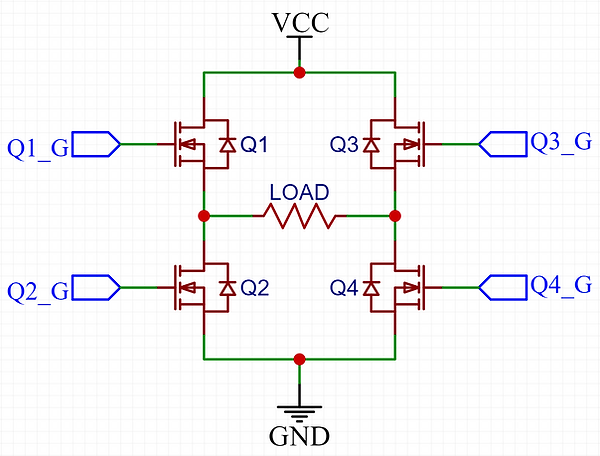Imagine Vcc being our supply voltage, say rectified mains which would give us 325VDC. Now imagine what would happen if we turned on transistors Q1 and Q4 simultaneously.In this setup the current flows through the load to the ground. Notice the direction of the current. Now let's turn off Q1 and Q4 transistors and let's turn on the diagonally opposing transistors Q3 and Q2.The current is now flowing through the load again but this time in the different direction. Now if we keep alternating between transistors Q1,Q4 and Q3,Q2 we will have an alternating current flow through our load. Since we can control the transistors with digital logic, we can drive them at any frequency we want, with some limitations of course as no transistor switches infinitely quick. This way we created an AC square wave voltage on our load from DC source. Such a circuit is called an inverter and you can find a similar circuit in any switch mode power supply all the way from a puny phone charger up to huge multi kilowatt server power supplies. Although usually the load is not a simple resistor but a coil of wire forming a primary of a transformer. H-bridge is a type of inverter circuit. There are many more different topologies with various electrical components to make up an inverter.

Now we could just measure the resonant frequency of the secondary and then program the driver to always oscillate on this frequency to drive the primary coil at resonance with the secondary, just like in this schematics:Well this would kind of work in a fixed surrounding, but the problem is that the environment around the secondary coil always changes. Even the arc itself adds a capacitance to the secondary circuit therefore lowering the secondary resonant frequency, thus making the driver oscillate at a wrong frequency. We usually solve this issue with some kind of feedback from the secondary coil such as a feedback transformer.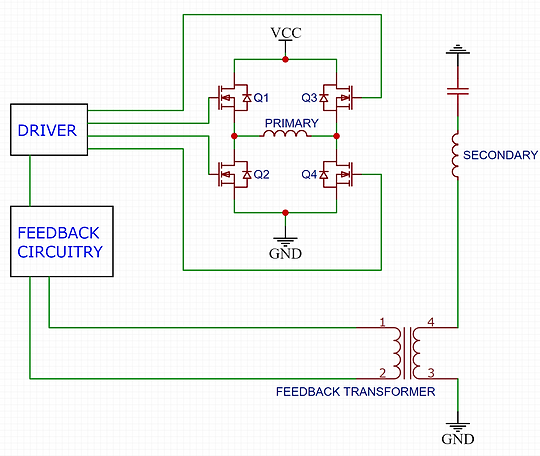Feedback transformer usually only consists of lower pole of the secondary being wired through a ferrite core transformer with N windings. Thus making a transformer with 1:N ratio. Some kind of feedback circuit is then utilized to adjust the frequency of the driver to oscillate at the secondary resonant frequency at all times. This way we can heavily change the resonant frequency of the secondary for example by drawing a huge arc straight to ground and still keeping the driver oscillating at resonance. This is a huge improvement from an SGTC which oscillates at a fixed frequency of the primary resonant circuit.6th August 2021

Notice that the primary circuit unlike in the SGTC is not a resonant circuit and it is not driven in resonance, only the secondary circuit is in resonance with the H-bridge. This has a few advantages as well as disadvantages. The main advantage here is that the primary coil acts as an inductive impedance which limits the flowing current and thus power draw. Remember how "long" were the oscillations in the SGTC? They usually are in the order of 100s of microseconds. But thanks to low power consumption of an SSTC we can run much longer pulses, we can actually go fully continuous if well designed. Or we can design our primary to have a very low impedance and feed in short high power pulses just like in an SGTC. With a solid state design, the possibilities are huge. We can even play clean audio through the sparks as we can easily change the driving frequency and thus modulate the output power. The obvious disadvantage here is that this kind of circuit is way more complicated than an SGTC and requires a lot of electronics knowledge to put it together. Also unlike a spark gap which is virtually indestructible, transistors are quite fragile components and can be easily damaged for example with an overvoltage or overtemperature.

Here are some examples of an SSTC7th August 2021Solid State Tesla coil made by KaizerPowerElectronics running interrupted modeSolid State Tesla coil made by DiodeGoneWild running without feedback circuitSolid State Tesla coil made by Loneoceans Laboratories running interrupted mode

Fully continuous SSTC made by me with clean audio modulation